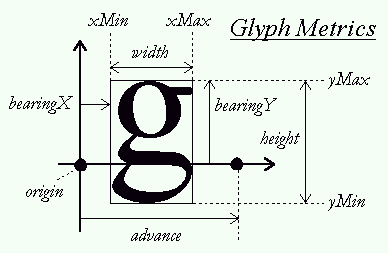DAY 23
0

• 載入字體檔(.ttf)

關於渲染字形這件事1. 字母與基準線的關係，例如: 小寫字母pj這些在基準線之下會有資料需要畫出(Bearing)
3. 曲線(這個有一大堆可以說，礙於本人能力不足，還沒研究過)

stb_truetype的使用

typedef struct CharInfo {
int unicode;
unsigned char* data;
Rect rec;
int xoffset, yoffset;
} CharInfo;

typedef struct Font {
Texture texture;
int base_height;
CharInfo chars[ASCII_CHAR_COUNT];
} Font;

/* prepare font */
stbtt_fontinfo info;
if (!stbtt_InitFont(&info, fontBuffer, 0))
{
printf("failed\n");
}

int b_w = 512; /* bitmap width */
int b_h = 128; /* bitmap height */
int l_h = 64; /* line height */

/* create a bitmap for the phrase */
unsigned char* bitmap = calloc(b_w * b_h, sizeof(unsigned char));

/* calculate font scaling */
float scale = stbtt_ScaleForPixelHeight(&info, l_h);

目前做了甚麼

stbrp_context* context = (stbrp_context*)malloc(sizeof(stbrp_context));
stbrp_node* nodes = (stbrp_node*)malloc(sizeof(ASCII_CHAR_COUNT * sizeof(stbrp_node)));

stbrp_init_target(context, font_atlas.w, font_atlas.h, nodes, ASCII_CHAR_COUNT);
stbrp_rect* rects = (stbrp_rect*)malloc(ASCII_CHAR_COUNT * sizeof(stbrp_rect));

for (int i = 0; i < ASCII_CHAR_COUNT; i++) {
rects[i].id = i;
rects[i].w = font->chars[i].rec.w + 2 * padding;
rects[i].h = font->chars[i].rec.h + 2 * padding;
}

stbrp_pack_rects(context, rects, ASCII_CHAR_COUNT);